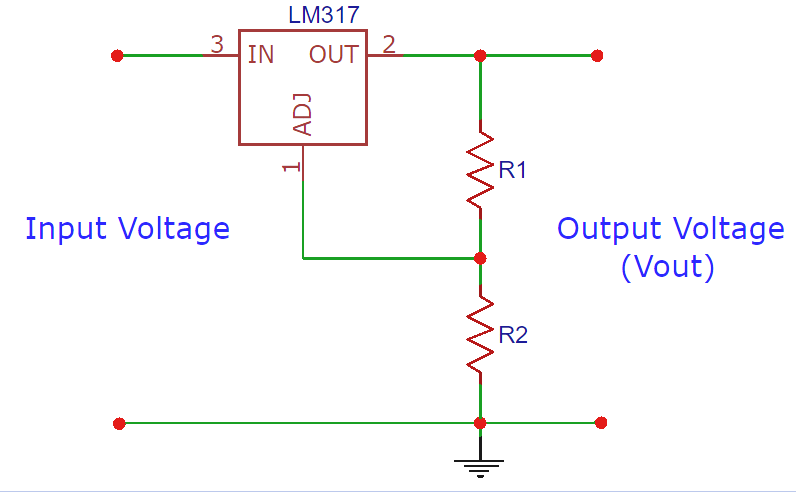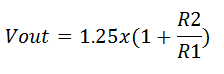# LM317 Resistor/Voltage Calculator

Enter the R1 and R2 resistor values in the below LM317 calculator to calculate the output voltage, OR you can enter the target output voltage and R1 and can calculate the required  value of R2 resistor.Resistance 1 (R1) OhmKilo OhmMega Ohm Resistance 2 (R2) OhmKilo OhmMega Ohm Output Voltage (Vout): Volt

### How LM317 Calculator works?

LM317 is an adjustable voltage regulator which takes an input voltage of 3 - 40V DC and provides a fixed output voltage of 1.25V to 37V DC. It requires two external resistors to adjust the output voltage.

The output voltage Vout is dependent on external resistor values R1 and R2, according to the following equation:The recommended value for R1 is 240Ω but it can also be some other value between 100Ω to 1000Ω. So you need to enter a value of R2 in the LM317 voltage calculator to calculate the output voltage. For example let’s take the R2 value of 1000Ω, so according to the formulae above the calculations for output voltage would be as follows:

Vout = 1.25x(1 + 1000/240) = 6.458V

Similarly if you have a target output voltage then you can calculate the R2 value using the LM317 formulae given above. For example if the target output voltage is 10V then the R2 value is calculated as following:

10 = 1.25x(1 + R2/240)

=> R2 = 1680Ω

So this is how we calculate the R2 and output voltage for LM317 voltage regulator circuit. This LM317 calculator can be also be used for some other ICs like LM338 or LM350.